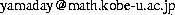Department of Mathematics, Kobe University
Rokko, 657-8501, JapanUpdated on: Apr. 13, 2015

### Papers on archives

• arXiv:1408.5643
From polygons to ultradiscrete Painleve equations

• arXiv:1405.2745
Quadrirational Yang-Baxter maps and the affine-E8 Painleve lattice

• arXiv:1302.6298
A Common Structure in PBW Bases of the Nilpotent Subalgebra of U_q(g) and Quantized Algebra of Functions

• arXiv:1206.5963
Symmetries of quantum Lax equations for the Painleve equations

• arXiv:1204.0294
Pade interpolation for elliptic Painleve equation

• arXiv:1011.0292
A quantum isomonodromy equation and its application to N=2 SU(N) gauge theory

• arXiv:1008.0574
Localization with a surface operator, Irregular conformal blocks and Open topological string

• arXiv:1004.5122
Five-dimensional AGT relation and the deformed beta-ensemble

• arXiv:1004.1687
Lax formalism for q-Painlev'e equations with affine Weyl group symmetry of type E^{(1)}_n

• arXiv:1001.1813
Generalized energies and integrable D^{(1)}_n cellular automaton

• arXiv:0910.4431
Five-dimensional AGT Conjecture and the Deformed Virasoro Algebra

• math:0811.1796
A lax formalism for the elliptic difference Painlev'e equation

• math.QA/0610505
Tau functions in combinatorial Bethe ansatz
A. Kuniba, R. Sakamoto, Y. Yamada

• math.QA/0601630
Crystal interpretation of Kerov-Kirillov-Reshetikhin bijection

• hep-th/0512092
Five-dimensional Supergravity and Hyperbolic Kac-Moody Algebra G2H
S. Mizoguchi, K. Mohri, Y. Yamada

• nlin.SI/0501051
Construction of Hypergeometric Solutions to the q-Painlev'e Equations
K. Kajiwara, T. Masuda, M. Noumi, Y. Ohta, Y. Yamada

• nlin.SI/0411044
Box-ball system with reflecting end

• nlin.SI/0411003
Point configurations, Cremona transformations and the elliptic difference Painlev'e equation
K. Kajiwara, T. Masuda, M. Noumi, Y. Ohta, Y. Yamada

• nlin.SI/0403009
Cubic Pencils and Painlev'e Hamiltonians
K. Kajiwara, T. Masuda, M. Noumi, Y. Ohta, Y. Yamada

• nlin.SI/0403036
Hypergeometric solutions to the q-Painlev'e equations
K. Kajiwara, T. Masuda, M. Noumi, Y. Ohta, Y. Yamada

• nlin.SI/0303032
$_10E_9$ solution to the elliptic Painlev'e equation
K. Kajiwara, M. Noumi, T. Masuda, Y. Ohta, Y. Yamada

• math.QA/0303088
Tropical R and Tau Functions

• math-ph/0203029
A new Lax pair for the sixth Painlev'e equation associated with $\hat{\mathfrak{so}}(8)$

• math-ph/0203030
Tropical Robinson-Schensted-Knuth correspondence and birational Weyl group actions

• math.QA/0208239
Geometric Crystal and Tropical R for D^(1)_n

• nlin.SI/0106029
Discrete dynamical systems with $W(A^{(1)}_{m-1} \times A^{(1)}_{n-1})$ symmetry
K. Kajiwara, M. Noumi, Y. Yamada

• math.QA/0109140
Difference L operators related to q-characters

• nlin.SI/0112045
q-Painlev'e systems arising from q-KP hierarchy
K. Kajiwara, M. Noumi, Y. Yamada

• math.QA/0007175
Scattering rules in soliton cellular automata associated with crystal bases

• math.QA/0012028
Birational Weyl group action arising from a nilpotent Poisson algebra

• nlin.SI/0012063
A study on the fourth q-Painlev'e equation
K. Kajiwara, M. Noumi, Y. Yamada

• hep-th/9907134
Affine 7-brane Backgrounds and Five-Dimensional $E_N$ Theories on $S^1$

• solv-int/9908007
Determinant Formulas for the Toda and Discrete Toda Equations
K. Kajiwara, T. Masuda, M. Noumi, Y. Ohta, Y. Yamada

• math.QA/9908116
Energy Functions in Box Ball Systems

• hep-th/9909122
Mordell-Weil Lattice via String Junctions
M. Fukae, Y. Yamada, S.-K. Yang

• math.QA/9802085
Character Formulae of $\hat{sl}_n$-Modules and Inhomogeneous Paths
G. Hatayama, A. N. Kirillov, A. Kuniba, M. Okado, T. Takagi, Y. Yamada

• math.QA/9804132
Affine Weyl groups, discrete dynamical systems and Painleve equations

• math.QA/9808002
Determinant formulas for the $\tau$-functions of the Painlev'e equations of type $A$

• math.QA/9808003
Higher order Painlev'e equations of type $A^{(1)}_l$

• math.QA/9812022
Remarks on Fermionic Formula

• q-alg/9702019
On $q$-Clebsch Gordan Rules and the Spinon Character Formulas for Affine $C_2^{(1)}$ Algebra

• q-alg/9708018
Symmetries in the fourth Painleve equation and Okamoto polynomials

• hep-th/9504052
Crystalizing the Spinon Basis

• hep-th/9505083
Crystalline Spinon Basis for RSOS Models

• q-alg/9512027
Kostka Polynomials and Energy Functions in Solvable Lattice Models

• hep-th/9405106
On the Genus Expansion in the Topological String Theory
T. Eguchi, Y. Yamada, S.-K. Yang

• hep-th/9302048
Topological Strings, Flat Coordinates and Gravitational Descendants
T. Eguchi, H. Kanno, Y. Yamada, S.-K. Yang

• hep-th/9304121
Topological Field Theories and the Period Integrals
T. Eguchi, Y. Yamada, S.-K. Yang

• hep-th/9306096
Elliptic Genera and N=2 Superconformal Field Theory
Toshiya Kawai, Y. Yamada, Sung-Kil Yang

• hep-th/9205002
Fusion Rules for the q-deformed Vertex Operators of U_q(sl(2)^)